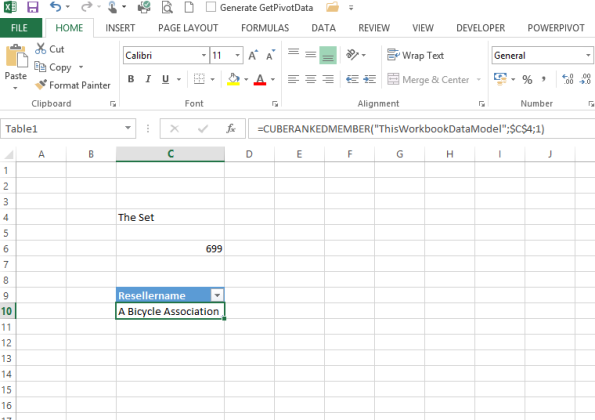### Cube Functions In Excel

Cube functions allow Excel to access data from an Analysis Services data source without using a Pivot Table. There are many different types of cube function:

## CUBEVALUE function

CubeValue function returns an aggregated value from the cube.

The syntax of the CUBE Function is:

=CUBEVALUE(connection, member_expression1, member_expression2…)

connection is a text string of the name of the connection to the cube

member_expression is a text string that evaluates to a member within the cube

Example:

=CUBEVAULE (“Sales”,” [Measures].[Profit]”, “[Time].”,” [All Product].[Beverages]”)

=CUBEVALUE (\$A\$1,” [Member].[Profit]”, D212,\$A23)## CUBEMEMBER function

CUBEMEMBER returns an element or a tuple from the cube.

The syntax of CUBEMEMBER function is:

=CUBEMEMBER (connection, member_expression, )

Connection is a text string of the name of the connection to the cube

member_expression is a text string that evaluates to a member within the cube

Caption is a text string that is displayed in the cell instead of the caption, if one is defined, from the cube.

Example:

=CUBEMEMBER (“Sales”, [months].[september])

## CUBEKPIMEMBER function

CUBEKPIMEMBER returns a key performance indicator (KPI) property and displays the KPI name in the cell

The syntax of CUBEKPIMEMBER is:

=CUBEKPIMEMBER (connection, kpi_name, kpi_property, )

Connection is a text string of the name of the connection to the cube

Kpi_name is a text string of the name of the kpi in the cube

Kpi_property is the Kpi component returned

Example:

=CUBEKPIMEMBER (“Sales”,”MySalesKPI”, 1)

=CUBEKPIMEMBER (“Sales”,”MySalesKPI”, KPIGoal,”Sales KPI Goal”)

## CUBERANKEDMEMBER function

Returns the nth, or ranked, member in a set.

Syntax

=CUBERANKEDMEMBER(connection, set_expression, rank, )

Examples

=CUBERANKEDMEMBER(“Sales”,\$D\$4,1,”Top Month”)

=CUBERANKEDMEMBER(“Sales”,CUBESET(“Sales”,”Summer”,”.[June]”,”.[July]”,”.August]”),3,”Top Month”)## CUBESET function

Defines a calculated set of members or tuples by sending a set expression to the cube on the server, which creates the set, and then returns that set to Microsoft Excel.

Syntax

CUBESET(connection, set_expression, , [sort_order], [sort_by])

Set_expression is A text string of a set expression that results in a set of members or tuples.

Caption is a text string that is displayed in the cell instead of the caption, if one is defined, from the cube.

Sort_order is the type of sort, if any, to perform

Examples

=CUBESET(“Finance”,”Order([Product].[Product].[Product Category].Members,[Measures].[UnitSales],ASC)”,”Products”)

=CUBESET(“Sales”,”Filter([Product].[Level_1].[Level_1].Members, Right([Product].[Level_1].CurrentMember.Name, 2)=””_Wisconsin””)”)

=CUBESET(“ThisWorkbookDataModel”,(A1,A2),”Product1 & Product2″)

## CUBESETCOUNT function

Returns the number of items in a set.

The syntax of the CUBESETCOUNT Function is

=CUBESETCOUNT(set)

Where Set is a text string of a Microsoft Excel expression that evaluates to a set defined by the CUBESET function.

Examples:

=CUBESETCOUNT (A3)It is possible to create formulas using nested cube functions. For example you can create something like:

=CUBEVALUE(“DataModel”,”MyMeasure”,”Date”,”MyFilter”,CUBESET(“DataModel”,”MyFilter2″))

## Template

```Further reading: